Search

Graphing Rational Functions:
The Special Case with the "Hole"
(page 4 of 4)

Sections: Introduction, Examples, The special case with the "hole"• Graph the following:
•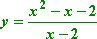First I'll find any vertical asymptotes, by setting the denominator equal to zero and solving:

x  2 = 0
x = 2

 So I have a vertical asymptote at x = 2. I'll dash this in: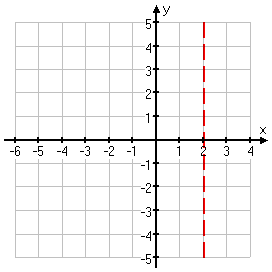Looking at the degrees of the numerator and denominator, I see that the numerator is a quadratic while the denominator is linear. Since the degree is one greater in the numerator, I know that I will have a slant asymptote. But I need to do the long division to find out what the equation of the slant asymptote is going to be.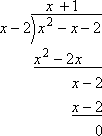Hmm... The slant asymptote is clearly y = x + 1, but there is no remainder; the division came out evenly. That's because the numerator happens to factor as (x  2)(x + 1), so the x  2 factor divides out evenly and there is no remainder.

 In any case, the slant (not horizontal) asymptote is at y = x + 1: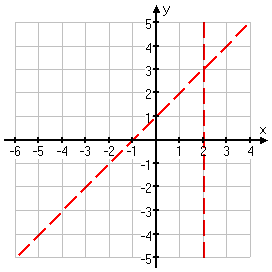x = 0:  y = (0  0  2)/(0  2) = 1

y = 0:  0 = (x2  x  2) / (x  2)

0 = x2  x  2

0 = (x  2)(x + 1)

x = 2, x = 1

 I know that I can't have x = 2, as an x-intercept, because this is actually the vertical asymptote. So I'll ignore that "solution" of the above equation. The intercepts then are (0, 1) and (1, 0):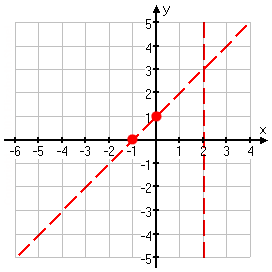Now I'll plot a few more points. However, since this example is actually a special case of slant-asymptote problem (and something of a trick question), I'll also show you the points for the slant asymptote only.

 x y = (x2  x  2)/(x  2) y = x + 1 6 y = (36 + 6  2)/(6  2) = 5 y = 5 5 y = (25 + 5  2)/(5  2) = 4 y = 4 4 y = (16 + 4  2)/(4  2) = 3 y = 3 3 y = (9 + 3  2)/(3  2) = 2 y = 2 2 y = (4 + 2  2)/(2  2) = 1 y = 1 1 y = (1 + 1  2)/(1  2) = 0 y = 0 0 y = (0  0  2)/(0  2) = 1 y = 1 1 y = (1  1  2)/(1  2) = 2 y = 2 2  y is not defined  y = 3 3 y = (9  3  2)/(3  2) = 4 y = 4 4 y = (16  4  2)/(4  2) = 5 y = 5

Do you see how that, except for the one point where the rational function isn't defined (at x = 2), the two lines are the same? In general, this is not true for rationals. But in this special case  where the long division works out with no remainder  you don't actually even need the asyptote lines dashed in. Instead, you find the slant asymptote equation (in this case, y = x + 1), and you draw that in for the rational graph.

 The only difference between the slant asymptote of the rational function and the rational function itself is that the rational function isn't defined at x = 2. To account for this, I leave a nice big open circle at the point where x = 2, showing that I know that this point is not actually included on the graph, because of the zero in the denominator of the rational.Warning: This graph is not the norm for rationals. But you should expect to encounter one like this, perhaps on the test. I've only ever seen this done with slant asymptotes, but it could be done with horizontal asymptotes, too. Keep in mind that you can't just "cancel off" duplicate factors when graphing rationals; you still need to account for all the zeroes of the denominator.

Note that your calculator will probably not show this hole in the graph, and "TRACE" will probably miss it too, since it is unlikely that x = 2 will be exactly on the pixel that the calculator is processing. But even if the graph does not show the gap in the line, the calculator will show no value for y when x is two.

 When I asked my calculator to evaluate y for x = 2, I got this: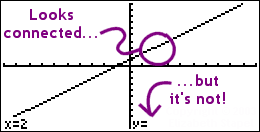If it just so happens that the vertical asymptote falls on a pixel (rather than between two pixels), then the gap in the graph will display on the calculator's pretty picture.

 For instance, when I changed the window settings on my graphing calculator so the x-values ran from 7 to 7 (rather than the default 10 to 10), the gap was (just barely) visible: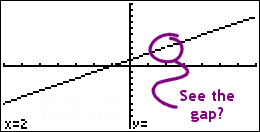When you do these graphs, get in the habit of working methodically through all the steps (vertical asymptote(s), horizontal or slant asymptote, intercept(s), plotted points, and graph), so you can do the graphing easily when you get to the test. And, as you can see above, you will need to know this material well enough that your calculator doesn't mess you up.

<< Previous  Top  |  1 | 2 | 3 | 4  |  Return to Index

 Cite this article as: Stapel, Elizabeth. "Graphing Rational Functions: The Special Case with the 'Hole'."     Purplemath. Available from https://www.purplemath.com/modules/grphrtnl4.htm.     Accessed [Date] [Month] 2016

Study Skills Survey

Tutoring from Purplemath
Find a local math tutor